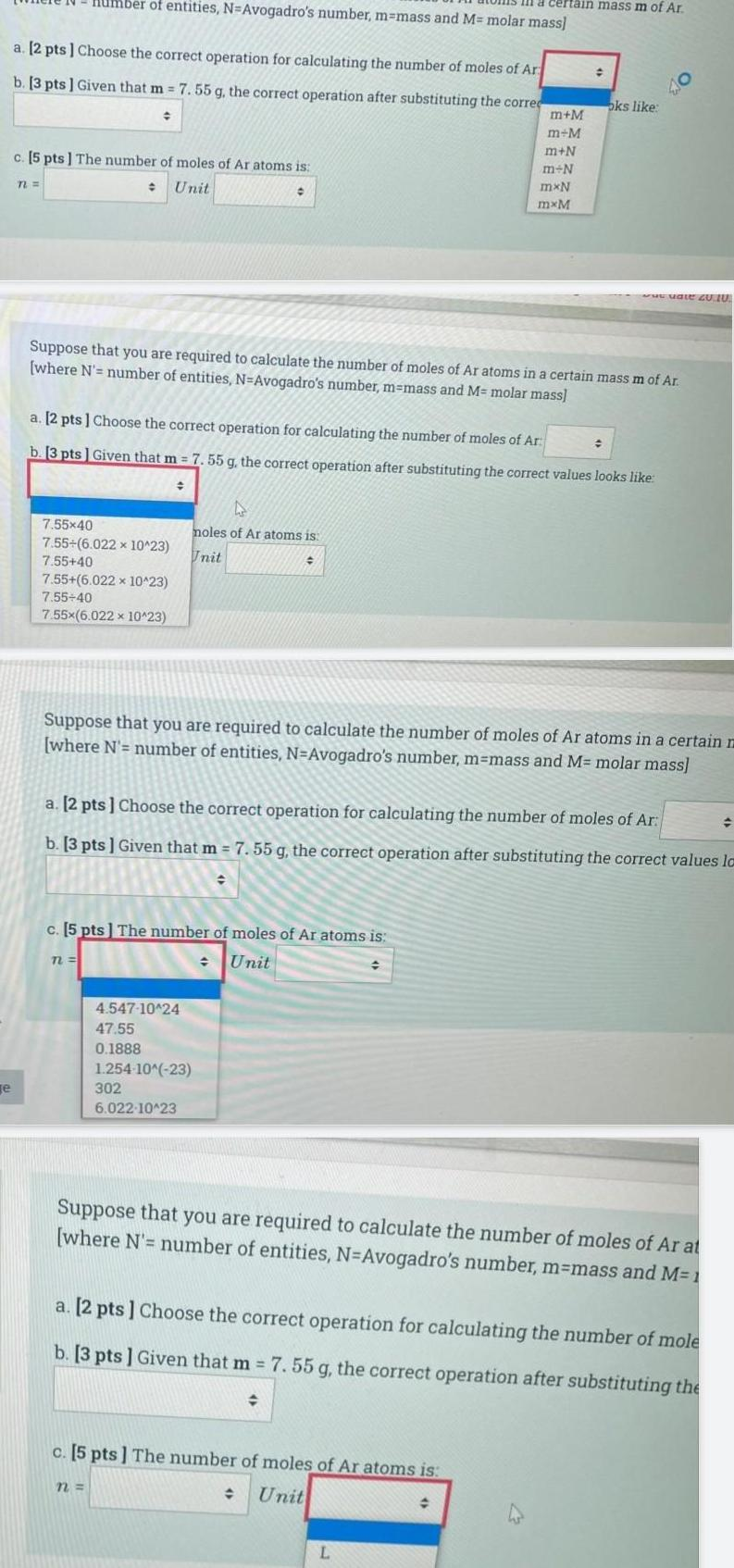Question:

# e a 2 pts Choose the correct operation for calculating the

Last updated: 7/4/2023e a 2 pts Choose the correct operation for calculating the number of moles of Ar b 3 pts Given that m 7 55 g the correct operation after substituting the correc c 5 pts The number of moles of Ar atoms is Unit n Imber of entities N Avogadro s number m mass and M molar mass 7 55x40 7 55 6 022 x 10 23 7 55 40 7 55 6 022 x 10 23 7 55 40 7 55x 6 022 x 10 23 72 Suppose that you are required to calculate the number of moles of Ar atoms in a certain mass m of Ar where N number of entities N Avogadro s number m mass and M molar mass a 2 pts Choose the correct operation for calculating the number of moles of Ar b 3 pts Given that m 7 55 g the correct operation after substituting the correct values looks like c 5 pts The number of moles of Ar atoms is Unit D moles of Ar atoms is Unit 4 547 10 24 47 55 0 1888 n 1 254 10 23 302 6 022 10 23 Suppose that you are required to calculate the number of moles of Ar atoms in a certain m where N number of entities N Avogadro s number m mass and M molar mass certain mass m of Ar a 2 pts Choose the correct operation for calculating the number of moles of Ar b 3 pts Given that m 7 55 g the correct operation after substituting the correct values la m M m M m N m N mxN mxM c 5 pts The number of moles of Ar atoms is Unit oks like L 40 www uate 20 10 Suppose that you are required to calculate the number of moles of Ar at where N number of entities N Avogadro s number m mass and M 1 a 2 pts Choose the correct operation for calculating the number of mole b 3 pts Given that m 7 55 g the correct operation after substituting the